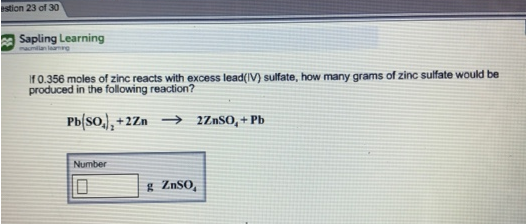# Problem: If 0,356 moles of zinc reacts with excess lead(V) sulfate, how many grams of zinc sulfate would be produced in the following reaction? Pb(SO4)2 + 2Zn → 2ZnSO4 + Pb

###### FREE Expert Solution
92% (463 ratings)###### Problem Details

If 0,356 moles of zinc reacts with excess lead(V) sulfate, how many grams of zinc sulfate would be produced in the following reaction?

Pb(SO4)2 + 2Zn → 2ZnSO4 + Pb